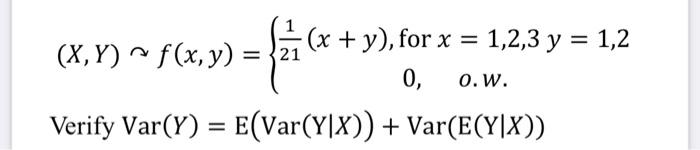Home / Expert Answers / Statistics and Probability / x-y-curvearrowright-f-x-y-left-begin-array-c-frac-1-21-x-y-text-for-x-1-2-3-pa323

# (Solved): $$(X, Y) \curvearrowright f(x, y)=\left\{\begin{array}{c}\frac{1}{21}(x+y), \text { for } x=1,2,3 ...\( (X, Y) \curvearrowright f(x, y)=\left\{\begin{array}{c}\frac{1}{21}(x+y), \text { for } x=1,2,3 y=1,2 \\ 0, \quad o . w .\end{array}\right.$$ $$\operatorname{Verify} \operatorname{Var}(Y)=\mathrm{E}(\operatorname{Var}(\mathrm{Y} \mid X))+\operatorname{Var}(\mathrm{E}(\mathrm{Y} \mid X))$$

We have an Answer from Expert